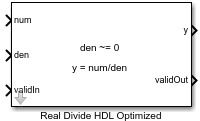# Real Divide HDL Optimized

Divide one real input by another and generate optimized HDL code

Since R2021a

•Libraries:
Fixed-Point Designer HDL Support / Math Operations

## Description

The Real Divide HDL Optimized block outputs the result of dividing the real scalar `num` by the real scalar `den`, such that `y` = `num`/`den`.

## Limitations

Data type override is not supported for the Real Divide HDL Optimized block.

## Ports

### Input

expand all

Numerator, specified as a real-valued scalar, vector, or matrix. `num` and `den` must have the same dimensions.

Slope-bias representation is not supported for fixed-point data types.

Data Types: `single` | `double` | `fixed point`

Denominator, specified as a real-valued scalar, vector, or matrix. `num` and `den` must have the same dimensions.

Slope-bias representation is not supported for fixed-point data types.

Data Types: `single` | `double` | `fixed point`

Whether input is valid, specified as a Boolean scalar. This control signal indicates when the data from the `num` and `den` input ports are valid. When this value is `1` (`true`), the block captures the values at the input ports `num` and `den`. When this value is `0` (`false`), the block ignores the input samples.

Data Types: `Boolean`

### Output

expand all

Output computed by dividing `num` by `den`, such that `y` = `num`/`den`, returned as a real scalar with the data type specified by the Output datatype parameter.

Data Types: `single` | `double` | `fixed point`

Whether the output data is valid, returned as a Boolean scalar. When the value of this control signal is `1` (`true`), the block has successfully computed the output at port `y`. When this value is `0` (`false`), the output data is not valid.

Data Types: `Boolean`

## Parameters

expand all

Data type of the output `y`, specified as `fixdt(1,18,10)`, `single`, `fixdt(1,16,0)`, or as a user-specified data type expression. The type can be specified directly or expressed as a data type object, such as `Simulink.NumericType`.

#### Programmatic Use

 Block Parameter: `OutputType` Type: character vector Values: `'fixdt(1,18,10)'` | `'single'` | `'fixdt(1,16,0)'` | ```''``` Default: `'fixdt(1,18,10)'`

## Tips

The blocks Divide by Constant HDL Optimized, Real Divide HDL Optimized, and Complex Divide HDL Optimized all perform the division operation and generate optimized HDL code.

• Real Divide HDL Optimized and Complex Divide HDL Optimized are based on a CORIDC algorithm. These blocks accept a wide variety of inputs, but will result in greater latency.

• Divide by Constant HDL Optimized accepts only real inputs and a constant divisor. Use of this block consumes DSP slices, but will complete the division operation in fewer cycles and at a higher clock rate.

expand all

## Version History

Introduced in R2021a